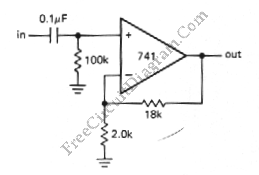# Noninverting Amplifier with Op-AmpThis is a noninverting amplifier circuit. This circuit amplify the input voltage with the same phase with output voltage. This circuit has infinite input impedance, but using 741, the input impedance would be hundreds of megaohms. The output impedance is a little, in fraction of an ohm. Here is the schematic diagram of the circuit:This circuit is made for a DC amplifier. To used with AC coupled input source, the input current must returned to the ground.The voltage gain of this circuit is determined by following equation:
gain = Vout/ Vin = 1 + R2/R1
Using a shown values, the voltge gain is 10 with a low-frequency 3dB point of 16Hz.